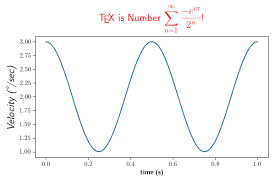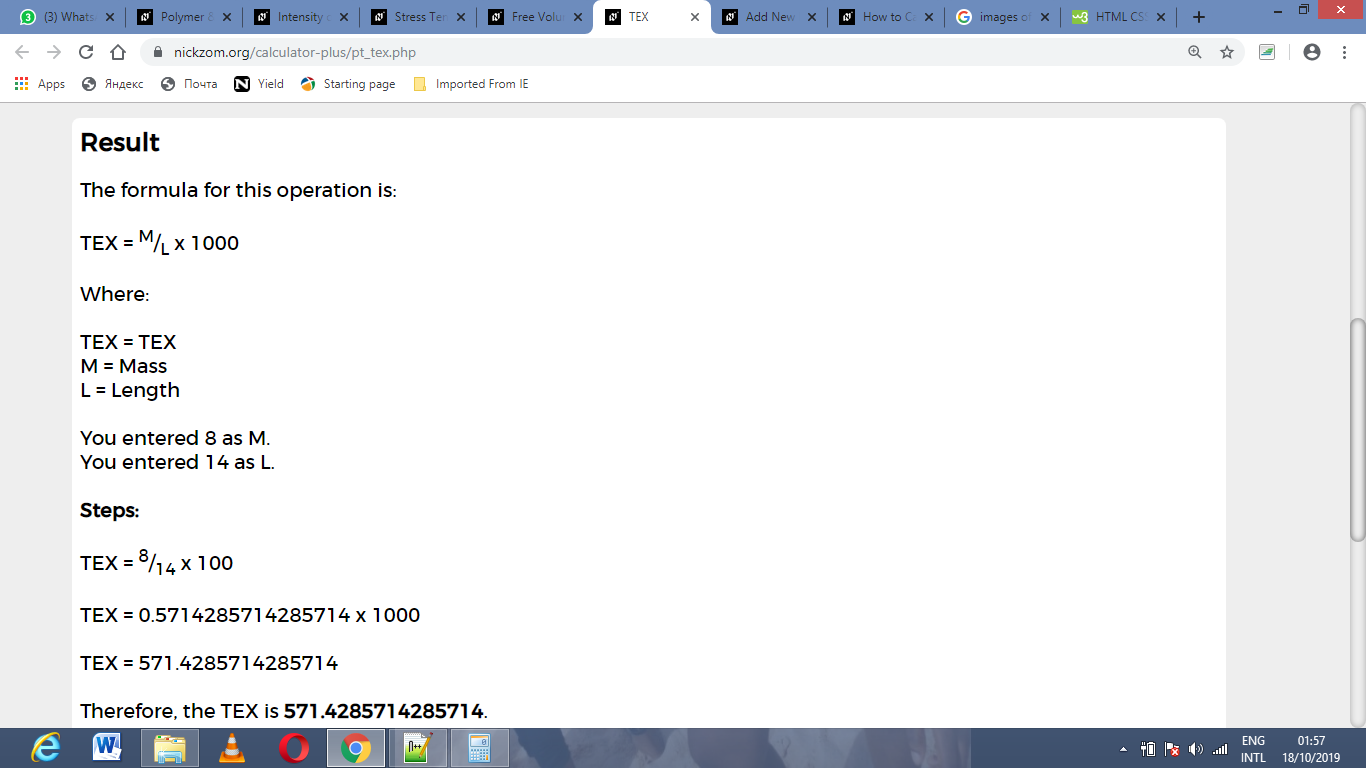# How to Calculate and Solve for TEX | Polymer & TextileThe image above represents TEX.

To compute for TEX, two essential parameters are needed and these parameters are mass (M) and length (L).

The formula for calculating TEX:

TEX = M/L x 1000

Where:

TEX = TEX
M = Mass
L = Length

Let’s solve an example;
Find the TEX when the mass is 8 and the length is 14.

This implies that;

M = Mass = 8
L = Length = 14

TEX = M/L x 1000
TEX = 8/14 x 1000
TEX = 0.57 x 1000
TEX = 571.4

Therefore, the TEX is 571.4.

Calculating the Mass when the TEX and the Length is Given.

M = TEX x L / 1000

Where:

M = Mass
TEX = TEX
L = Length

Let’s solve an example;
Find the mass when the TEX is 40 and the length is 8.

This implies that;

TEX = TEX = 40
L = Length = 8

M = TEX x L / 1000
M = 40 x 8 / 1000
M = 320 / 1000
M = 0.32

Therefore, the mass is 0.32.

Calculating the Length when the TEX and the Mass is Given.

L = M1000 / TEX

Where;

TEX = TEX
M = Mass

Let’s solve an example;
Find the length with a TEX of 50 and a mass of 20.

This implies that;

TEX = TEX = 50
M = Mass = 20

L = M1000 / TEX
L = (20)1000 / 50
L = 20000 / 50
L = 400

Therefore, the length is 400.

Nickzom Calculator – The Calculator Encyclopedia is capable of calculating the TEX.

To get the answer and workings of the TEX using the Nickzom Calculator – The Calculator Encyclopedia. First, you need to obtain the app.

You can get this app via any of these means:

To get access to the professional version via web, you need to register and subscribe for NGN 1,500 per annum to have utter access to all functionalities.
You can also try the demo version via https://www.nickzom.org/calculator

Apple (Paid) – https://itunes.apple.com/us/app/nickzom-calculator/id1331162702?mt=8
Once, you have obtained the calculator encyclopedia app, proceed to the Calculator Map, then click on Polymer & Textile under Engineering.Now, Click on TEX under Polymer & TextileThe screenshot below displays the page or activity to enter your values, to get the answer for the TEX according to the respective parameters which is the mass (M) and length (L).Now, enter the values appropriately and accordingly for the parameters as required by the mass (M) is 8 and length (L) is 14.Finally, Click on CalculateAs you can see from the screenshot above, Nickzom Calculator– The Calculator Encyclopedia solves for the TEX and presents the formula, workings and steps too.